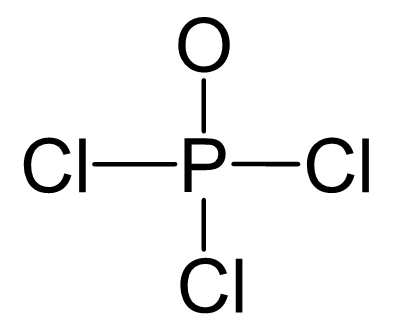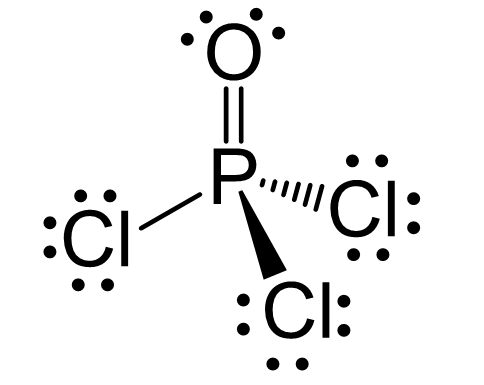## Examples

P is the central atom, so we can draw a preliminary skeletal structure:There are 5 + 3×7 + 6 = 32 valence electrons out of which, 8 are already taken to make four covalent bonds. From the remaining 24, 18 go to the Cl atoms as three lone pairs per each, three 6 to the oxygen as three lone pairs. This leaves the central atom with 4 atoms connected to it and no lone pairs. Therefore, both geometries are tetrahedral.Steric number 4 corresponds to sp3-hybridization where the idealized bond angles are 109.5o. Because the groups on the central atom are not identical, the bond angles differ from the idealized values.

Check Also